Computer Science And IT (CS/IT) Mock Test For Gate

# Computer Science And IT (CS/IT) Mock Test For Gate

Test Description

## 65 Questions MCQ Test GATE Computer Science Engineering(CSE) 2023 Mock Test Series | Computer Science And IT (CS/IT) Mock Test For Gate

Computer Science And IT (CS/IT) Mock Test For Gate for Computer Science Engineering (CSE) 2023 is part of GATE Computer Science Engineering(CSE) 2023 Mock Test Series preparation. The Computer Science And IT (CS/IT) Mock Test For Gate questions and answers have been prepared according to the Computer Science Engineering (CSE) exam syllabus.The Computer Science And IT (CS/IT) Mock Test For Gate MCQs are made for Computer Science Engineering (CSE) 2023 Exam. Find important definitions, questions, notes, meanings, examples, exercises, MCQs and online tests for Computer Science And IT (CS/IT) Mock Test For Gate below.
Solutions of Computer Science And IT (CS/IT) Mock Test For Gate questions in English are available as part of our GATE Computer Science Engineering(CSE) 2023 Mock Test Series for Computer Science Engineering (CSE) & Computer Science And IT (CS/IT) Mock Test For Gate solutions in Hindi for GATE Computer Science Engineering(CSE) 2023 Mock Test Series course. Download more important topics, notes, lectures and mock test series for Computer Science Engineering (CSE) Exam by signing up for free. Attempt Computer Science And IT (CS/IT) Mock Test For Gate | 65 questions in 180 minutes | Mock test for Computer Science Engineering (CSE) preparation | Free important questions MCQ to study GATE Computer Science Engineering(CSE) 2023 Mock Test Series for Computer Science Engineering (CSE) Exam | Download free PDF with solutions
 1 Crore+ students have signed up on EduRev. Have you?
Computer Science And IT (CS/IT) Mock Test For Gate - Question 1

### In the following question, some part of the sentence may have errors. Find out which part of the sentence has an error and select the appropriate option. If the sentence is free from error, select 'No error'.The fox thought to him The fox thought to him (A)/ that he should wait for the (B)/ lady to leave the place.(C)/ No error

Detailed Solution for Computer Science And IT (CS/IT) Mock Test For Gate - Question 1

The error lies in part (A) of the sentence as the pronoun ‘him’ is incorrect and must be replaced with a reflexive pronoun ‘himself’ which is used as the object of a verb or preposition to refer to a male person or animal previously mentioned as the subject of the clause.

Computer Science And IT (CS/IT) Mock Test For Gate - Question 2

### A book shelf has 27 books – 9 books each written by 3 eminent authors. If Raj selects any 2 books without booking at their author’s name, what is the probability that he picks up books written by the different authors.

Detailed Solution for Computer Science And IT (CS/IT) Mock Test For Gate - Question 2

Total possible outcomes  = 27C2

Favourable outcomes = Selecting any two authors × selecting one book for each author=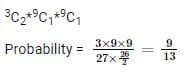Computer Science And IT (CS/IT) Mock Test For Gate - Question 3

### The ratio material cost and labor cost in a small construction project is 3:1. If the labor cost went up by 20% and material cost witnessed an avenge increase of 15%, what will be the new ratio of labor cost and material cost?

Detailed Solution for Computer Science And IT (CS/IT) Mock Test For Gate - Question 3

Let us take material cost and labor cost as Rs 300 and Rs 100 respectively. Since labor cost went up by 20%, the revised labor cost is Rs 120. The revised material cost due to 15% increase is Rs (300+15% of 300) = 345. The ratio of labor cost and material cost is 120: 345 or 8: 23 i.e. (b)

Computer Science And IT (CS/IT) Mock Test For Gate - Question 4

A monkey is trying to reach the top of a tree. He climbs 3 feet in a minute but slides down by 1.5 feet after each climb. If he reaches the top of the tree in 58 minutes, then the maximum possible height of the tree is ____ feets.

Detailed Solution for Computer Science And IT (CS/IT) Mock Test For Gate - Question 4

You have to carefully observe the point that in the last minute, we DO NOT have to consider the sliding down of the monkey since the question states that the monkey reaches the top of the tree.Out of 58 minutes, the climb by monkey in 58th minute is 3 feet whereas for each of his earlier climbs, the monkey covers a net distance of 3-1.5=1.5  feet.We can work out the maximum possible height of the tree as 1.5×57+3=85.5+3=88.5 feet

Computer Science And IT (CS/IT) Mock Test For Gate - Question 5

Direction: Read the sentence to find out whether there is any error in it. The error, if any, will be in one part of the sentence. If the given sentence is correct as it is, mark E i.e. No error as the answer. Ignore the errors of punctuation, if any.The misuse of drugs and alcohol were seen as very damaging/ to the society, chiefly because it often promoted/ violent crimes and anti-social behavior; while also/ causing ill-health, poverty and family breakdown.

Detailed Solution for Computer Science And IT (CS/IT) Mock Test For Gate - Question 5

The error lies in the first part of the sentence where the verb ‘were’ is incorrect and needs to be replaced with ‘was’ as the subject "misuse" is singular. To make the subject and the verb agree it should read as:’The misuse of drugs and alcohol was seen as..’

Computer Science And IT (CS/IT) Mock Test For Gate - Question 6

An event A is considered to be rolling a fair dice .If 10 dice are rolled each of which are independent, find the probability that the sum obtained on the faces is between 30 and 40 both inclusive.

Given: N(1.0184) = 0.84 , which is value of normal distribution for X = 1.018

Detailed Solution for Computer Science And IT (CS/IT) Mock Test For Gate - Question 6

Let Xi denote the ith dice.

Since events are independent,

E(xi) = 7/2

V(xi) = 350/12

According to Central Limit Theorem,

P(30≤ X ≤40) = P(30 – 35 ≤ X – 35 ≤ 40-35)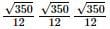Its converting to standard form, X – E[X] / std, where std is standard deviation.

Thus,

P = 2N(1.0184) – 1

= 0.69

Computer Science And IT (CS/IT) Mock Test For Gate - Question 7

Which of the given option is most appropriate in the context of the word in bold letters in the given sentence.The reason there is no exodus towards banks in general for new project funding is their relatively less experience in project appraisal.

Detailed Solution for Computer Science And IT (CS/IT) Mock Test For Gate - Question 7

Migration is the only word which comes to the meaning of the word ‘exodus.’

Computer Science And IT (CS/IT) Mock Test For Gate - Question 8

Improve the bracketed part of the sentence. My car (broke off) on my way to the office.

Detailed Solution for Computer Science And IT (CS/IT) Mock Test For Gate - Question 8

Let's understand the meanings of each phrasal verb given in the options:

Broke off = became separated/detached

Broke out = started/began suddenly

Broke in = force entry to a building

Broke down = suddenly ceased or stopped to function (of a machine or motor vehicle)

As per the context of the sentence, the correct phrasal verb is "broke down".

Computer Science And IT (CS/IT) Mock Test For Gate - Question 9

Chinnaswamy is driving to pick up his son from school on a Saturday which is a half day. On his way to school, he crossed a church which is 1/5th of the way to school at 9:50 hours and exactly 10 minutes later, he went past a temple which is 1/3rd of the way to school. The time after 10:00 hours at which he reaches his son’s school is ____ minutes.

Detailed Solution for Computer Science And IT (CS/IT) Mock Test For Gate - Question 9

Since we are given time to cross a point which is 1/5th of the way and 1/3rd of the way, we can rewrite them as 3/15th and 5/15th of the way which implies that 2/15th of the way was covered in 10 minutes.
This means that the entire route was covered in 10 × 15/2 = 75 minutes (each 1/15th part is covered in 75/15 = 5 minutes) leading to time of arrival at school as 9:50 – 15 + 75 = 10:50 hours.

Computer Science And IT (CS/IT) Mock Test For Gate - Question 10

In the following question, out of the four alternatives, select the word similar in meaning to the given word.Literal

Detailed Solution for Computer Science And IT (CS/IT) Mock Test For Gate - Question 10

Literal = line for line, letter to letter
Verbatim = in exactly the same words; word for word
Idealistic = visionary, dreamy, unrealistic
Outdated = out of date, obsolete
Hence, "Verbatim" best expresses the meaning of the word "Literal".

Computer Science And IT (CS/IT) Mock Test For Gate - Question 11

In control flow analysis, control flow graph contain basis blocks for the code.

A basic block is a segment of the code that a program must enter at the beginning and it can exit only at the end. A basic block ends in which of the following ways?

Detailed Solution for Computer Science And IT (CS/IT) Mock Test For Gate - Question 11

A basic block can end in any of the following:Jump statementConditional/ unconditional branchReturn statement

Computer Science And IT (CS/IT) Mock Test For Gate - Question 12

Which of the following operators is used to search a specified pattern in a column?

Detailed Solution for Computer Science And IT (CS/IT) Mock Test For Gate - Question 12

The like operator is used in the string to search for characters. Eg: All names starting with ‘A’ (select name from ABC were name like ‘A%’;) while where is used to check for the condition.

Computer Science And IT (CS/IT) Mock Test For Gate - Question 13

Draw the process state transition diagram of an OS in which (i) each process is in one of the five states: created, ready, running, blocked (i.e. sleep or wait), or terminated, and (ii) only non-preemptive scheduling is used by the OS. Label the transitions appropriately.

Detailed Solution for Computer Science And IT (CS/IT) Mock Test For Gate - Question 13

The following typical process states are possible on computer systems of all kinds. In most of these states, processes are "stored" on main memory.
Created: When a process is first created, it occupies the "created" or "new" state. Ready and waiting: A "ready" or "waiting" process has been loaded into main memory and is awaiting execution on a CPU. There may be many "ready" processes at any one point of the system's execution.
Running: A process moves into the running state when it is chosen for execution. The process's instructions are executed by one of the CPUs (or cores) of the system. There is at most one running process per CPU or core. A process can run in either of the two modes, namely kernel mode or user mode.
Kernel mode : Processes in kernel mode can access both: kernel and user addresses.Kernel mode allows unrestricted access to hardware including execution of privileged instructions.
User mode : Processes in user mode can access their own instructions and data but not kernel instructions and data .access to any hardware device is allowed.
Blocked : A process that is blocked on some event (such as I/O operation completion or a signal), may be blocked due to various reasons, such as exhausting its CPU time allocation or waiting for an event to occur.
Terminated : A process may be terminated, either from the "running" state by completing its execution or by explicitly being killed

Computer Science And IT (CS/IT) Mock Test For Gate - Question 14

An instruction is stored at location 300 with its address field at location 301. The address field has the value 400. A processor register R1 contains the number 200. What will be the addressing mode of the effective address of the instruction is 702?

Detailed Solution for Computer Science And IT (CS/IT) Mock Test For Gate - Question 14

Relative: 302 + 400 = 702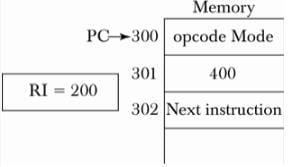Computer Science And IT (CS/IT) Mock Test For Gate - Question 15

A file system is said to exhibit data dependence because;

Detailed Solution for Computer Science And IT (CS/IT) Mock Test For Gate - Question 15

A file system is said to exhibit data dependence because a change in any file’s structure, such as addition or deletion of a field, requires modifications of all programs using that file. That means, access to a file is dependent on its structure.
A file system is said to exhibit data dependence because a change in any file’s data characteristics, such as changing the filed from integer to decimal, requires change in all data access programs. That means, access to a file data is dependent on its data characteristics.

Computer Science And IT (CS/IT) Mock Test For Gate - Question 16

Choose the correct option to fill the empty blanks in below statement :If variables are declared previously, then __________ is responsible for generation of symbol table but if variables are not declared then symbol table will be generated by __________.

Detailed Solution for Computer Science And IT (CS/IT) Mock Test For Gate - Question 16

If variables are declared previously, then semantic analyzer is responsible for generation of symbol table.

If variables are not declared, then lexical analyzer will generate the symbol table.

Hence option b is correct.

Computer Science And IT (CS/IT) Mock Test For Gate - Question 17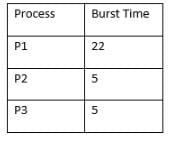Above process arrive in the order P1, P2, P3 and are served in FCFS order. Then average waiting time is:

Detailed Solution for Computer Science And IT (CS/IT) Mock Test For Gate - Question 17

Gantt chart for the given situation is: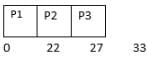Now, the waiting time is 0 milliseconds for process P1, 22 unit for process P2 and 27 unit for process P3

Thus, the average waiting time = (0 + 27 + 22)/ 3 = 49/3 = 16.33 = 16 units.

Computer Science And IT (CS/IT) Mock Test For Gate - Question 18

Initially a priority queue had 5 elements and it is being implemented as Max-Heap. Let’s say the level order traversal of the heap is: 30, 18, 17, 12, 11. Now two more elements are inserted into the heap i.e. 10 and 19 in the same order. The level order traversal of the heap after the insertion is:

Detailed Solution for Computer Science And IT (CS/IT) Mock Test For Gate - Question 18

So, the insertion is followed in the same order, hence, we will insert 10 first which doesn’t effect the tree. Now, we insert 19. After insertion of 19 max_heapify founds an error in the node 17 → 11, 17 → 19. So, 19 is send up and 17 is brought down. After that the level order traversal changes to 30, 18, 19, 12, 11, 10, 17

Computer Science And IT (CS/IT) Mock Test For Gate - Question 19

Create a view which gives students number and name for those who enrolled in class ”Database Design” and whose age is under 25.

Detailed Solution for Computer Science And IT (CS/IT) Mock Test For Gate - Question 19

Following is the syntax for creating views.

CREATEVIEW view_name AS

SELECT column_name(s)

FROM table_name

WHERE condition

Computer Science And IT (CS/IT) Mock Test For Gate - Question 20

Consider the following C program:

int main(){

printf("what %%");

return 0;

What does the code print?

Detailed Solution for Computer Science And IT (CS/IT) Mock Test For Gate - Question 20

Backslash actually acts an escape character and helps to print double quotes, where % can be printed only with double % i.e. %%

Computer Science And IT (CS/IT) Mock Test For Gate - Question 21

Consider a program which stores the frequency of marks of student in a particular subject say Mathematics. The marks is in the range [0...100] and there are around 500 students. But there is one condition we only want to record frequency if the student has passed in the exam, the passing marks being 40. What is the best way to store the frequency of marks above 40?

Detailed Solution for Computer Science And IT (CS/IT) Mock Test For Gate - Question 21

For this purpose, we can ignore the below forty numbers and have an array of 60 index to store the individual frequencies from 40 to 100.

*Answer can only contain numeric values
Computer Science And IT (CS/IT) Mock Test For Gate - Question 22

A fair die with faces {1, 2, 3, 4, 5, 6} is thrown repeatedly till ‘3’ is observed for the first time. Let X denote the number of times the die is thrown. The expected value of X is _____.

Detailed Solution for Computer Science And IT (CS/IT) Mock Test For Gate - Question 22

given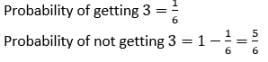now, the random variable X does represent number of throws required for getting 3. So,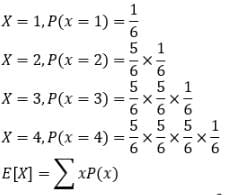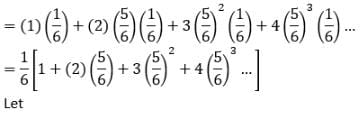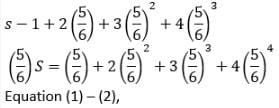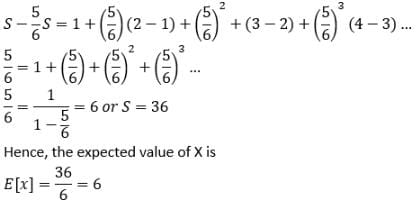Computer Science And IT (CS/IT) Mock Test For Gate - Question 23

The alternate way of writing the instruction, SUB #7,R1 is______. The above addressing mode is Immediate addressing mode

Detailed Solution for Computer Science And IT (CS/IT) Mock Test For Gate - Question 23

The SUBI instruction, means the subtraction is in immediate addressing mode.

Computer Science And IT (CS/IT) Mock Test For Gate - Question 24

Which one of the following is a closed form expression for the generating function for the sequence {Sn}, where Sn = an, for all n = 0, 1, 2, …… and ‘a’ is a constant?

Detailed Solution for Computer Science And IT (CS/IT) Mock Test For Gate - Question 24

The given sequence is <1, a1, a2, a3, a4, ………..>.

The generating function for this sequence will be;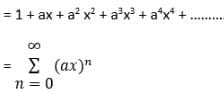The only generating sequence that matching the above sequence is of option D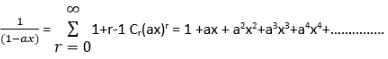Computer Science And IT (CS/IT) Mock Test For Gate - Question 25

Given two complex numbers,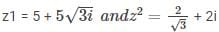the argument of z1/z2 in degrees is

Detailed Solution for Computer Science And IT (CS/IT) Mock Test For Gate - Question 25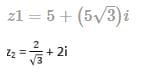since , we know that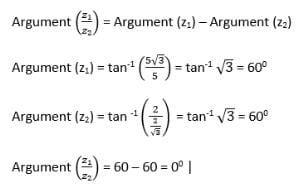Since we know that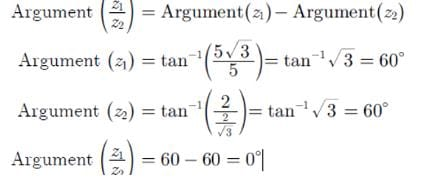Computer Science And IT (CS/IT) Mock Test For Gate - Question 26

An athlete can play on several teams and each team needs atleast one player.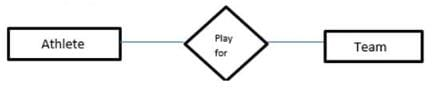Based on the description above, what is the maximum cardnality between each instance of “Athelete” and “team”?

Detailed Solution for Computer Science And IT (CS/IT) Mock Test For Gate - Question 26

As it is clearly mentioned that each team at least require one player so one team can acquire n number of athletes where n>=1. And also one athlete can play for more than one team i.e for m teams. So maximum cardinality is m:n

Computer Science And IT (CS/IT) Mock Test For Gate - Question 27

Find the type of given grammar:

S -> #S | @ A#

A -> @ A @ | #B!A

B -> &B% | ! | e

Non Terminal (V) = { S , A , B }

Terminal (T) = { # , ! , @ , % , & }

Here e is epsilon or null symbol.

Detailed Solution for Computer Science And IT (CS/IT) Mock Test For Gate - Question 27

To check LL(1) we have to use some rules :
i. First(A1) ∩ First(A2) ∩ ...... ∩ First(An) = Ø
Where , A -> A1 | A2 | A3 ...... | An
ii. If any production contains null then do as follow
First(Ai) ∩ Follow(Ai) = Ø
If the above two rules satisfy then given grammar is LL(1) , but as per grammar given in question it is not satisfying above rule in production of B [ First(B) ∩ Follow(B) != Ø ].
To check LR(0) when we draw DFA for each item in Goto and Clousre , there is shift and reduce conflict.
Same case is with SLR(1) , in production B , there is shift reduce conflict.

Computer Science And IT (CS/IT) Mock Test For Gate - Question 28

What is the unit digit of number obtained from: 111283+171293 - 71283

Detailed Solution for Computer Science And IT (CS/IT) Mock Test For Gate - Question 28

We don’t need to calculate the final result, just calculate the unit digit raised to the power of unit digit of power of each term and add it. So,  1+7-3=5

Computer Science And IT (CS/IT) Mock Test For Gate - Question 29

Consider the following system of equations.

x+y = 2

x+py = 2

where p is constant. Find the value of ‘p’ such that the system has more than two solutions.

Detailed Solution for Computer Science And IT (CS/IT) Mock Test For Gate - Question 29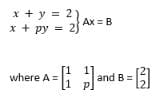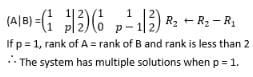Computer Science And IT (CS/IT) Mock Test For Gate - Question 30

When number of entities are bought together into one entity based on their similar characteristics, what is this process called;

Detailed Solution for Computer Science And IT (CS/IT) Mock Test For Gate - Question 30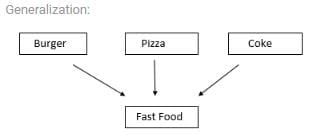In the above example food items are generalized on the basis that they all belong to fast food.

Computer Science And IT (CS/IT) Mock Test For Gate - Question 31

Let S be the reference string and X, Y be the no. Of page fault occur using the optimal and LRU page replacement policies respectively. Let SR be the reverse of S, and X' and Y' be the no. Of page fault occur using the optimal and LRU page replacement policies respectively. Which of the following is true?

Detailed Solution for Computer Science And IT (CS/IT) Mock Test For Gate - Question 31

It is the property of Optimal and LRU page replacement that S and SR will have same number of page fault.

Also, FIFO suffer from Belady’s anomaly but LRU and Optimal do not suffer from belady’s anomaly.

Computer Science And IT (CS/IT) Mock Test For Gate - Question 32

Consider 4-block cache (initially empty) with the following main memory block references.
4, 5, 7, 12, 4, 5, 13, 4, 5, 7
Identify the hit ratio for Direct mapped cache?

Detailed Solution for Computer Science And IT (CS/IT) Mock Test For Gate - Question 32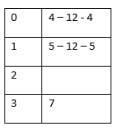{K MOD N = i}

4-M: 4 MOD 4 = 0

5-M: 5 MOD 4 = 1

7-M: 7 MOD 4 = 3

12-M: 12 MOD 4 = 0

4-M; 4 MOD 4 = 0

5-H

13-M; 13 MOD 4 = 1

4-H

5-M; 5 MOD 4 = 1

7-H

H = 3/10 = 0.3

Computer Science And IT (CS/IT) Mock Test For Gate - Question 33

Find the output

#include <iostream>

#define cnct(x,y) x##y

using namespace std;

class {

public:

int p,q,pq;

int f1()

{

cout<<p+q+pq+cnct(p,q)<<endl;

return (p+++q);

}

}c;

int main(){

c.p=5,c.q=6,c.pq=15;

cout<<c.f1();

return 0;

}

Detailed Solution for Computer Science And IT (CS/IT) Mock Test For Gate - Question 33

41, 11

Class without name is allowed. In this case object of class is created along with definition.

cnct(x,y) x##y ,## is a preprocessor macro used for concatenation.

x##y= xy

Call this creates a single terms named xy,here in main pq; cnct(p,q) places value of pq in its position that is 15.

cout<<p+q+pq+cnct(p,q)

5+6+15+15=41

P+++q is interpreted here as p++ + q(1st two for post increment operator,3rd one operator for adding )

Plus operator(+) has higher precedence than post increment operator. So, Value of p & q (5 & 6) get added before p increments. So, p+++q->11

Computer Science And IT (CS/IT) Mock Test For Gate - Question 34

The height of a tree is the length of the longest root to leaf path in it. The maximum and minimum number of nodes in a binary tree of height 4 is.

Detailed Solution for Computer Science And IT (CS/IT) Mock Test For Gate - Question 34

Height is defined as path length i.e (height+1) will give number of levels. In a binary tree of height h = 4;

Maximum number of nodes = 2^(h+1)-1

= 31

Minimum number of nodes = 5

Computer Science And IT (CS/IT) Mock Test For Gate - Question 35

The five aggregations operators in SQL are;

Detailed Solution for Computer Science And IT (CS/IT) Mock Test For Gate - Question 35

SQL uses the five aggregations operators SUM, AVG, MIN, MAX and COUNT. These operators are used by applying them to a scalar-valued expressions, typically a column name, in a SELECT clause.

Computer Science And IT (CS/IT) Mock Test For Gate - Question 36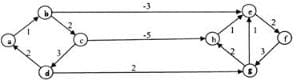Dijkstra’s single source shortest path algorithm when run from vertex a in the above graph, computes the correct shortest path distance to;

Detailed Solution for Computer Science And IT (CS/IT) Mock Test For Gate - Question 36

Dijkstra's single source shortest path is not guaranteed to work for graphs with negative weight edges, but it works for the given graph.

Let us see...

Let us run the 1st pass b 1 b is minimum, so shortest distance to b is 1

After 1st pass, distances are c 3. e -2. e is minimum, so shortest distance toe is -2 After 2nd pass, distances are c 3, f 0. f is minimum, so shortest distance t of is 0 After 3rd pass, distances are c 3, g 3. Both are same, let us take g. so shortest distance to g is 3. After 4th pass, distances are c 3, h 5 c is minimum, so shortest distance to c is 3 After 5th pass, distances are h -2 h is minimum, so shortest distance to h is -2.

*Answer can only contain numeric values
Computer Science And IT (CS/IT) Mock Test For Gate - Question 37

Consider a company, where a team is having seven different tasks. We have to complete these tasks with only four employees. Among these task, one task is tougher than other tasks. Similarly, we have one employee that is better than among other employees. In how many ways we can seven different tasks be assigned to four different employees so that each employee is assigned at least one task and the most difficult task is assigned to the best employee is _____________?

Detailed Solution for Computer Science And IT (CS/IT) Mock Test For Gate - Question 37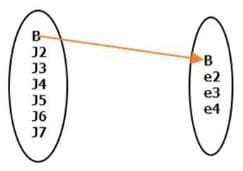As seen in the above image, best job is already assign to the best employee. Now we have 6 remaining job, that we need to assign to 4 employee. why 4?
Because best employee still can do other jobs.
Let N(e2) denotes no. of function where e2 is not assigned to any job.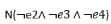this will represent the no. of functions where e2, e3 and e4 is assign to any job.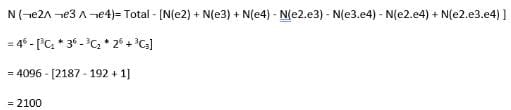why we are not counting best employee for this counting because best employee is always assign to the job

Computer Science And IT (CS/IT) Mock Test For Gate - Question 38

Consider the following function

int BSR (int arr[ ], int k)

{

int val=0, i, last;

for(i=0;i<k;i++)

value +=arr[i];

last =(k*(k-1))/2;

return (value-last);

}

Assume that the array contains list of all numbers from 1 to k-1.What is return value of function BSR?

Detailed Solution for Computer Science And IT (CS/IT) Mock Test For Gate - Question 38

This program finds a repeated number (duplicate) in the list. This program adds all values in the variable “ value” and substract “k(k-1)2” from it.Array has 0 to (k-1) locations and numbers are from 1 to (k-1).so one number will be repeated in the array.

Computer Science And IT (CS/IT) Mock Test For Gate - Question 39

Assuming that a pointer take 4 bytes and the size of an integer is 2 bytes. What is the size of the *a in declaration: int (*a)  ?

Detailed Solution for Computer Science And IT (CS/IT) Mock Test For Gate - Question 39

When you dereference a pointer, it actually gives out the type of object being pointed. Hence, in this case it is int and the answer is 10 * 2 * 2 (integer size ) = 40

Computer Science And IT (CS/IT) Mock Test For Gate - Question 40

Consider the following logical inferences

(A) “If you have a current password, then you can log onto the network.”

Inference: “You can log onto the network.”

(B) “If Sita to go school then Ram will go to school.”

“Sita doesn’t go to school”.

Inference: “Ram doesn’t go to school”

Which of the following is TRUE?

Detailed Solution for Computer Science And IT (CS/IT) Mock Test For Gate - Question 40

Inference A, is Modus Ponens.

Inference B, is false.

Let P = Sita go to school.

Q = Ram go to school.

For P →Q to be true.

If P is true then Q has to be true. (There is no other choice for Q).

But if P is false then Q can be anything (True or False). Still P →Q is true.

This is also known as "The fallacy of denying the antecedent".

P →Q

~P

∴(Nothing can be concluded).

Computer Science And IT (CS/IT) Mock Test For Gate - Question 41

The complex number  z = log (3+4i) in the standard form is;

Detailed Solution for Computer Science And IT (CS/IT) Mock Test For Gate - Question 41

The given expression can be written in a+ib form as :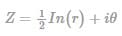where r, θ are the magnitude and argument of z respectively.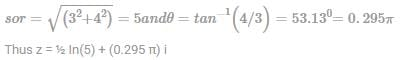Computer Science And IT (CS/IT) Mock Test For Gate - Question 42

Consider the following function.

Void rajkumar(int n)

{

enqueue(Q,0);

enqueue(Q,1);

for (i=0;i<n;i++)

{

x = dequeue(Q);

y = dequeue(Q);

enqueue(O,y);

enqueue(Q+x+y);

print(x);

}

}

What is the functionality of above function rajkumar?

Detailed Solution for Computer Science And IT (CS/IT) Mock Test For Gate - Question 42

The function prints first n fibbonacci numbers note that 0 and 1 are initially there in the queue. In every iteration of loop, sum of two queue items is enqueued and the front item is dequeued.

Computer Science And IT (CS/IT) Mock Test For Gate - Question 43

Consider the following graph.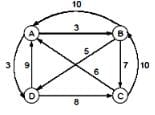Assume starting vertex is A. What is the cost of optimal tour by travelling salesperson using the dynamic programming?

Detailed Solution for Computer Science And IT (CS/IT) Mock Test For Gate - Question 43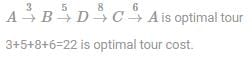Computer Science And IT (CS/IT) Mock Test For Gate - Question 44

How many bit strings of length 8 are possible that either start with a 1 or end with 00?

Detailed Solution for Computer Science And IT (CS/IT) Mock Test For Gate - Question 44

Total number of bit strings that start with two zeros (26) or end in a one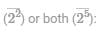26+27−25

Computer Science And IT (CS/IT) Mock Test For Gate - Question 45

Assume a matrix A[-5….+5][5…50]  is stored in a linear in row major order. Base address of A is 0 and each element takes 1 word. Find the location of A[–2] .

Detailed Solution for Computer Science And IT (CS/IT) Mock Test For Gate - Question 45

+[(-2+5)*(50-5+1)+(50+5)]*1

= 0+3*46+45=183

Computer Science And IT (CS/IT) Mock Test For Gate - Question 46

What is the maximum number of Binary Search Trees possible with 6 nodes?

Detailed Solution for Computer Science And IT (CS/IT) Mock Test For Gate - Question 46

Number of BST with ‘n’ nodes is given by 2nCn/(n+1)

=>12C6/7 =132.

Computer Science And IT (CS/IT) Mock Test For Gate - Question 47

Which of the following statements is not correct for a square matrix A? (| A | ≠ 0)

Detailed Solution for Computer Science And IT (CS/IT) Mock Test For Gate - Question 47

if we get A2 = I →then it's know as  Involuntary Matrix

if we get A2 = A → then matrix is Idempotent Matrix

and

If A is a symmetric matrix, A-1 will also be a symmetric matrix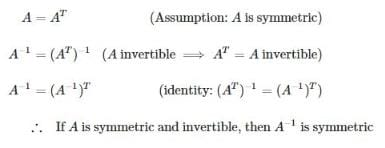Computer Science And IT (CS/IT) Mock Test For Gate - Question 48

Which is the correct option that shows minimization of figure shown?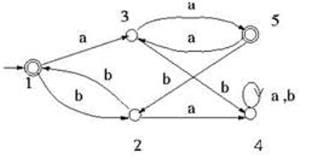Detailed Solution for Computer Science And IT (CS/IT) Mock Test For Gate - Question 48

In this, we see that on b state, state 2 will go to state 1 with state 3 going to state 4 which further will go as state 1 and state 4 are in different set. Further, states 2 and 3 are different from each other. As state 4 goes to state 4 with state 3 goes to state 5 and state 4 which lies in different sets, hence state 3 and state 4 gets separated from each other. Further, state 2 goes to state 1 while state 4 goes to state 4 and to state 1. Further it is seen that state 2 and state 4 are separated from each other.

Further it is observed that as state 1 goes to state 3 placed on node a and further to state 2 which lies on node b as shown, hence the minimized DFA transitions are added from state 1 to state 3 on a while state 1 to state 2 on node b. Further as state 2 goes to state 1 on node b and state 3 goes to state 1 on node a, so minimized DFA transitions adds from state 2 to state 1 on node b and further state 3 to state 1 on node a.

Computer Science And IT (CS/IT) Mock Test For Gate - Question 49

Consider the relation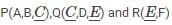having tuples 200, 300 and 100 respectively. Estimate number of tuples in relation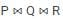Detailed Solution for Computer Science And IT (CS/IT) Mock Test For Gate - Question 49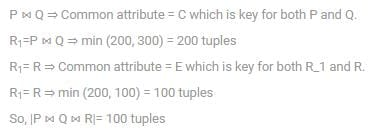Computer Science And IT (CS/IT) Mock Test For Gate - Question 50

Consider the following code snippet:

Do(int w, int x)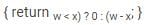Where w and x are any two positive integers. The call Do(30,do(30,90)) will return ;

Detailed Solution for Computer Science And IT (CS/IT) Mock Test For Gate - Question 50

Ternary operator has the following syntax:

exp 1 ? exp 2 : exp 3

if exp 1 is TRUE, then exp 2 is evaluated else exp 3 would be evaluated. Do(30,90)would return 0 and Do(30,0) would return 30.

Hence A) is the correct answer.

Computer Science And IT (CS/IT) Mock Test For Gate - Question 51

Let X ϵ {0, 1} and Y ϵ {0, 1} be two independent binary random variables. If P (X = 0) = p and P(Y = 0) = q, then P(X + Y ≥ 1) is equal to;

Detailed Solution for Computer Science And IT (CS/IT) Mock Test For Gate - Question 51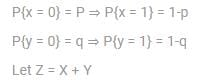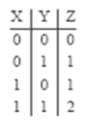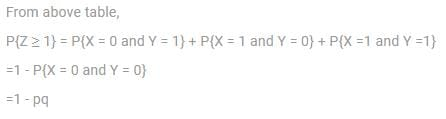Computer Science And IT (CS/IT) Mock Test For Gate - Question 52

A key that is chosen by the database designer as the principal means of identifying entities within an entity set is called a;

Detailed Solution for Computer Science And IT (CS/IT) Mock Test For Gate - Question 52

An attribute or set of attributes which can be used to distinguish every tuple in a table is called a super key.A super key for which no proper subset is a super key is called candidate key.There can be many candidate keys for a table and the candidate key selected by the database designer to identify the tuples uniquely is called a primary key.

Computer Science And IT (CS/IT) Mock Test For Gate - Question 53

Which cardinality best describes relationship runs where all team has drag experiment to runs?

Detailed Solution for Computer Science And IT (CS/IT) Mock Test For Gate - Question 53

From the above cardinalities, we see that ratios like 1:1, 1:N, N:1 and N:M gives cardinality constraint or numeric restriction on possible relationships. From this, we see that the ratio 1:N relation is most comfortable to describe the constant of ER team.

Computer Science And IT (CS/IT) Mock Test For Gate - Question 54

Consider a binary max-heap implemented using an array. Which of the following array represent a binary max-heap?

Detailed Solution for Computer Science And IT (CS/IT) Mock Test For Gate - Question 54

Max heap is stored in the array such that

Arr[k]>=Arr[2k] and Arr[k]>=Arr[2k+1]

Where k is the index of the array such that 1<=k<=n/2, Arr[2k] is left child and Arr[2k+1] is right child. Option (c) is the only array which satisfies above condition.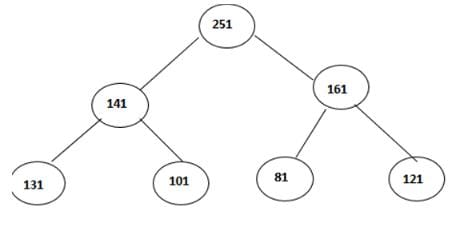Computer Science And IT (CS/IT) Mock Test For Gate - Question 55

Consider of the following propositional statements?

¬p∧(p→q→¬q
(p∧(p→q))→q
p→q)∧(q→r→(p→r)
(p∧(p→q))→¬q
Which of them are not valid?

Detailed Solution for Computer Science And IT (CS/IT) Mock Test For Gate - Question 55

1 is false because for P →Q to be true.

If P is true then Q has to be true. (There is no other choice for Q).

But if P is false then Q can be anything (True or False). Still P → Q is true.

This is also known as "The fallacy of denying the antecedent".

P → Q

~P

∴ (Nothing can be concluded).

2 is modus ponens so it is valid, similarly 3 is hypothetical syllogism, it is also valid.

4 is false because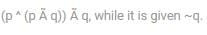Computer Science And IT (CS/IT) Mock Test For Gate - Question 56

Consider the following graph: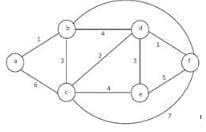Which one of the following cannot be the sequence of edges added, in that order, to a minimum spanning tree using Kruskal’s algorithm?

Detailed Solution for Computer Science And IT (CS/IT) Mock Test For Gate - Question 56

The edge (d-e) cannot be considered before (d-c) in Kruskal’s minimum spanning tree algorithm because Kruskal’s algorithm picks the edge with minimum weight from the current set of edges at each step.

Computer Science And IT (CS/IT) Mock Test For Gate - Question 57

Which of the following is illegal statement?

Detailed Solution for Computer Science And IT (CS/IT) Mock Test For Gate - Question 57

a) p is a function that takes an argument as an array of pointers to characters and returns a pointer to an integer.

b) p is a function that takes an argument as a pointer to a character array and returns an integer.

c) p is a pointer to a function that takes an argument as a pointer to a character and returns an integer.

d) This is an illegal statement.

Hence (d) is the correct answer

Computer Science And IT (CS/IT) Mock Test For Gate - Question 58

Consider two functional dependency set F and G: F={ A→B, B→C, C→A} and G= {A→BC, B→AC, AB→C, BC→A} which of the following is true?

Detailed Solution for Computer Science And IT (CS/IT) Mock Test For Gate - Question 58

Here, According to FD set F,

A+= {ABC}, B+= {ABC}, C+= {ABC}

According to FD set G,

A+= {ABC}, B+= {ABC}, C+= {C}

So, F covers G but G doesn’t covers F. Hence, option A is correct.

Computer Science And IT (CS/IT) Mock Test For Gate - Question 59

Which of the following statement is false?

Detailed Solution for Computer Science And IT (CS/IT) Mock Test For Gate - Question 59

(a)f(n)=n^2 logn(true)

(b) If the graph has no edges (True)

(c) In a DAG the strongly-connected components consist of single nodes. To compute them just

scan the array of nodes. (True)

(d) They form a tree but the nodes in this tree are only those nodes reachable by a path from ‘s’and there may be strictly less than ‘n’ such nodes, therefore the tree may have strictly less

than (n – 1) edges. (False)

Computer Science And IT (CS/IT) Mock Test For Gate - Question 60

There are x number of identical balls which are to be placed in y number of distinct buckets. If x>= ky (where k is a natural number greater than equal to 1), then in how many ways can you place the balls in the bucket with the condition that each bucket should contain at least k balls?

Detailed Solution for Computer Science And IT (CS/IT) Mock Test For Gate - Question 60

As there have to be at least k balls in each bag, so firstly put k balls in each bag i.e k*y balls. Balls remaining: x-ky

We can now apply balls and sticks method.

y bags= y variables, they need to equal to x-k*y, no restrictions on how many balls in each bag. We can make a equation out of this:

a1 + a2 + ... + ay = x- k*y

On solving it we get our answer:  (x-ky+y-1) C (y-1)

*Answer can only contain numeric values
Computer Science And IT (CS/IT) Mock Test For Gate - Question 61

A system that uses a two-level page table has 212 - byte pages and 36-bit virtual addresses. The first 8-bits of the address serve as the index into the first level page table.

The number of bits specify the second level index is ________?

Detailed Solution for Computer Science And IT (CS/IT) Mock Test For Gate - Question 61

as it is given that page size is 212 Bytes, so 12 bits are needed to specify that offset. The number of bits specify the second level index is:

36 - (12 + 8)

= 36 - 20

= 16

Computer Science And IT (CS/IT) Mock Test For Gate - Question 62

Consider the following rec function.

rec(int x)

{

static int f;

if (x==1)

return(1);

else

f+=x*rec(x-1);

return(f);

}

Find the value returned by rec(5).

Detailed Solution for Computer Science And IT (CS/IT) Mock Test For Gate - Question 62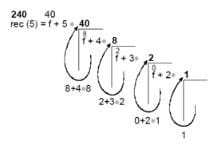Returning from x = 1 to 5 “f” value is updated being a static variable = 40 + 5 × 40 = 240.

Computer Science And IT (CS/IT) Mock Test For Gate - Question 63

What is the complexity of the efficient algorithm to construct a balanced BST from a given normal BST?

Detailed Solution for Computer Science And IT (CS/IT) Mock Test For Gate - Question 63

Most efficient solution would be to traverse given BST in inorder sequence and store in an array which takes O(n). Building a balanced BST from an array takes O(n). So complexity = O(n).

Computer Science And IT (CS/IT) Mock Test For Gate - Question 64

Consider the directed graph shown in the figure below. There are multiple shortest paths between vertices S and T. Which one will be reported by Dijkstra’s shortest path algorithm? Assume that, in any iteration, the shortest path to a vertex v is updated only when a strictly shorter path to v is discovered.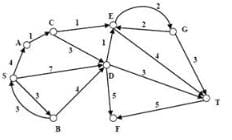Detailed Solution for Computer Science And IT (CS/IT) Mock Test For Gate - Question 64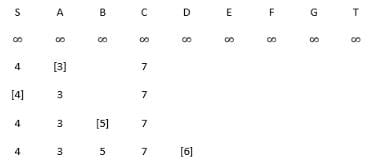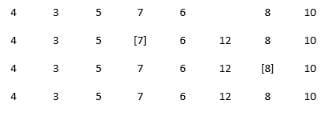Computer Science And IT (CS/IT) Mock Test For Gate - Question 65

Consider the following table with 4 processes: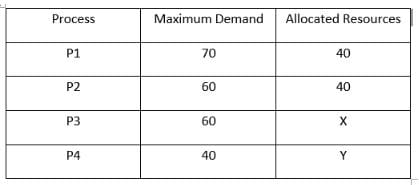If the system is having 150 unit of resources, Identify the value of X and Y for which the system will not be safe?

Detailed Solution for Computer Science And IT (CS/IT) Mock Test For Gate - Question 65

For the system not to be in safe state, values should be distributed in such a way that remaining resources should not be able to satisfy the need of any of the process.

After Observing, it is clear that when X= 40 and Y=20 the remaining need of the processes can be listed as: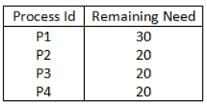Remaining Resources= 150-140=10

And we cannot satisfy the need of any of the given process.

Hence option d is the correct answer.

## GATE Computer Science Engineering(CSE) 2023 Mock Test Series

150 docs|215 tests
 Use Code STAYHOME200 and get INR 200 additional OFF Use Coupon Code
Information about Computer Science And IT (CS/IT) Mock Test For Gate Page
In this test you can find the Exam questions for Computer Science And IT (CS/IT) Mock Test For Gate solved & explained in the simplest way possible. Besides giving Questions and answers for Computer Science And IT (CS/IT) Mock Test For Gate, EduRev gives you an ample number of Online tests for practice

## GATE Computer Science Engineering(CSE) 2023 Mock Test Series

150 docs|215 tests